Courses

# Short Answer Type Questions: Circles Class 10 Notes | EduRev

## Class 10 : Short Answer Type Questions: Circles Class 10 Notes | EduRev

The document Short Answer Type Questions: Circles Class 10 Notes | EduRev is a part of the Class 10 Course Mathematics (Maths) Class 10.
All you need of Class 10 at this link: Class 10

Q1. Prove that the tangents drawn at the ends of a chord of a circle make equal angles with the chord.

Sol.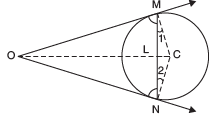Let NM be a chord of a circle with centre C.
Let the tangents at M and N meet at O.
∵ OM is a tangent at M

∵ ∠OMC = 90° ...(1)
Similarly ∠ONC = 90° ...(2)
Since, CM = CN [Radii of the same circle]
∵ In D CMN, ∠1= ∠2
From (1) and (2), we have
∠OMC –∠1 = ∠ONC –∠2
⇒ ∠OML = ∠ONL

Thus, tangents make equal angles with the chord.

Q2. Two concentric circles have a common centre O. The chord AB to the bigger circle touches the smaller circle at P. If OP = 3 cm and AB = 8 cm then find the radius of the bigger circle.

Sol. ∵ AB touches the smaller circle at P.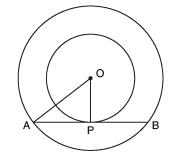∴ OP ⊥ AB ⇒ ∠OPA = 90°
Now, AB is a chord of the bigger circle.
Since, the perpendicular from the centre to a chord, bisects the chord,
∴ P is the mid-point of AB

⇒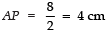In right ∆ APO, we have
AO= OP2 + AP2
⇒ AO2 = 32 + 42
⇒ AO2 = 9 + 16 = 25 = 52

⇒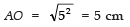Thus, the radius of the bigger circle is 5 cm.

Q3. In the given figure, O is the centre of the circle and PQ is a tangent to it. If its circumference is 12π cm, then find the length of the tangent.

Sol. ∵ Circumference of the circle = 12π cm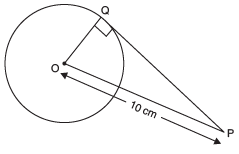∴ 2π r =12π
[∵ r is the radius of the circle]

⇒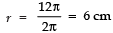⇒ Radius of the circle = 6 cm = OQ

Since a tangent to circle is perpendicular to the radius through the point of contact,
∴ ∠OQP = 90°
Now, in rt Δ OQP, we have:
OQ2 + QP2 = OP2
⇒ 62 + QP2 =102
⇒ QP2 = 102 − 62 = (10 − 6) (10 + 6) = 4 × 16 = 64 = 82

⇒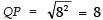Thus, the length of the tangent is 8 cm.

Q4. Given two concentric circles of radii 10 cm and 6 cm. Find the length of the chord of the larger circle which touches the other circle.

Sol. The chord AB touches the inner circle at P.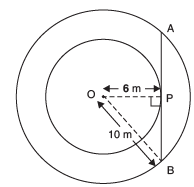∴ AB is tangent to the inner circle.
⇒ OP ⊥ AB

[∵ O is the centre and OP is radius through the point of contact P]
∴ ∠OPB = 90°.
Now, in right ∆ OPB, we have:
OP2 + PB2 = OB2
⇒ 62 + PB2 = 102
⇒ PB2 = 102 − 62
= (10 − 6) × (10 + 6)
⇒ PB2 = 4 × 16
⇒ PB2 = 64 = 82

⇒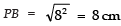∵ The radius perpendicular to a chord bisects the chord.
∴ P is the mid-point of AB
∴ AB = 2 × PB = 2 × 8 = 16 cm.

Q5. Two tangents TP and TQ are drawn to a circle with centre O from an external point T. Prove that ∠PTQ = 2 ∠OPQ.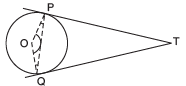Sol. ∵ Tangent to a circle is perpendicular to the radius through the point of contact.
∠OPT + ∠OQT + ∠POQ + ∠PTQ = 360°
or 90° + 90° + ∠POQ + ∠PTQ = 360°
⇒ ∠POQ + ∠PTQ = 360° − 90° − 90° = 180°   ...(1)
In Δ OPQ, ∠1 + ∠2 + ∠POQ = 180°   ...(2)

Since OP =OQ   [Radii of the same circle]
⇒ ∠1 = ∠2    [Angles opposite to equal sides]
∴  ∠OPT = 90° = ∠OQT
∴ From (2), we have
∠1 + ∠1 + ∠POQ = 180°
⇒ 2 ∠1 + ∠POQ = 180° ...(3)
From (1) and (3), we have
2 ∠1 + ∠POQ = ∠POQ + ∠PTQ
⇒ 2 ∠1= ∠PTQ
⇒ 2 ∠OPQ = ∠ PTQ.

Q6. In the figure, the incircle of ∆ ABC touches the sides BC, CA and AB at D, E and F respectively. If AB = AC, prove that BD = CD.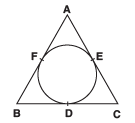Sol. Since the lengths of tangents drawn from an external point to a circle are equal,
∴ We have
AF = AE
BF = BD
CD = CE
(AF + BF) + CD = (AE + CE) + BD
⇒ AB + CD = AC + BD
But AB = AC  (Given)
∴ CD = BD.

Q7. A circle is touching the side BC of a D ABC at P and touching AB and AC produced at Q and R. Prove that: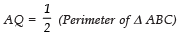Sol. Since, the two tangents drawn to a circle from an external point are equal.
∵  AQ = AR ...(1)
Similarly, BQ = BP ...(2)
and CR = CP ...(3)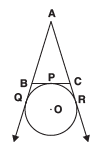Now, Perimeter of Δ ABC
= AB + BC + AC
= AB + (BP + PC) + AC
= AB + (BQ + CR) + AC
[From (2) and (3)]
= (AB + BQ) + (CR + AC)
= AQ + AR
= AQ + AQ [From (1)] = 2AQ
⇒Q8. In the given figure, the radii of two concentric circles are 13 cm and 8 cm. AB is diameter of the bigger circle. BD is the tangent to the smaller circle touching it at D. Find the length AD.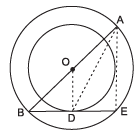Sol. Join AE and OD
∵ ∠ODB = 90°
[BE is a tangent at D and OD is a radius] and ∠AEB = 90° [AB is diameter so ∠AEB is an angle in semicircle so ∠AEB = 90°]
∴ OD ║ ΑΕ and ∆BEA are similar by AA-Similarity

So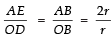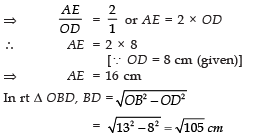Again in rt  Δ AED,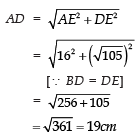Q9. In two concentric circles, a chord of the larger circle touches the smaller circle. If the length of this chord is 8 cm and the diameter of the smaller circle is 6 cm, then find the diameter of the larger circle.

Sol. Let the common centre be O. Let AB be the chord of the larger circle.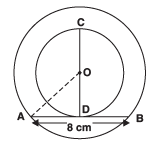∴ AB = 8 cm

And CD is the diameter of the smaller circle i.e., CD = 6 cm

⇒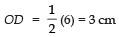Join OA. D is the point of contact.
∴ OD ⊥ AB
⇒ D is the mid point of AB
= 42 + 32 = 16 + 9 = 25 = 52
⇒ AO = 5 cm
⇒ 2AO = 2(5 cm) = 10 cm

∴ The diameter of the bigger circle is 10 cm.

Q10. In the following figure, PA and PB are two tangents drawn to a circle with centre O, from an external point P such that PA = 5 cm and ∠APB = 60°. Find the length of chord AB.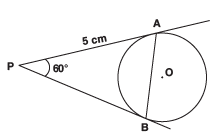Sol. Since the tangents to a circle from an external point are equal,
∴ PA = PB = 5 cm
In ΔPAB, we have
∠PAB = ∠PBA               [∵ PA = PB]
∴∠PAB + ∠PBA + ∠APB = 180°
⇒∠PAB + ∠PAB + 60° = 180°
⇒ 2 ∠PAB + 60° = 180°
⇒ 2 ∠PAB = 180°− 60°
= 120°
⇒ ∠PAB = 60°
⇒ Each angle of ∆PAB is 60°.
⇒ ΔPAB is an equilateral triangle. ∴
PA = PB
= AB = 5 cm
Thus, AB = 5 cm

Q11. In the following figure, AB is a chord of length 9.6 cm of a circle with centre O and radius 6 cm.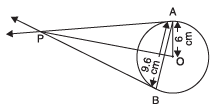The tangents at A and B intersect at P. Find the length PA.

Sol.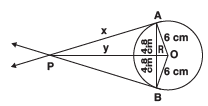Join OB.
Let PA = x cm and PR = y cm Since, OP is perpendicular bisector of AB

∴ AR = BR = 9.6/2 = 4.8 cm

Now, in rt ∆OAR, we have:
OA2 = OR2 + AR2
[By Pythagoras theorem]
⇒ OR2 = OA2 − AR2
= 62 − (4.8)2 = (6 − 4.8) × (6 + 4.8) = 1.2 × 10.8
⇒ = 12.96
OR = 3.6 cm.
Again, in right ΔOAP,
OP2 = AP2 + OA2
OP2 = (AR2 + PR2) + OA2
[∵ AP2 = AR2 + PR2]
⇒ (y + 3.6)2 = (4.8)2 + y2 + 62
⇒y2 + 12.96 + 7.2 y = 23.04 + y2 + 36
⇒ 7.2 y = 46.08

⇒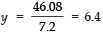⇒ PR = 6.4 cm
Now, AP2 = AP2 + PR2
= (4.8)2 + (6.4)2 = 23.04 + 40.96 = 64

⇒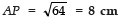Q12. Two tangents PA and PB are drawn to a circle with centre O from an external point P. Prove  that ∠APB = 2∠OAB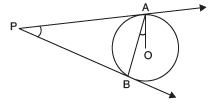Sol. We have PA and PB, the tangents to the circle and O is the centre of the circle.
∴ PA = PB
⇒∠2 = ∠4  ...(1)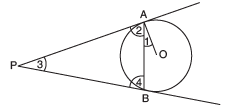Since the tangent is perpendicular to the radius through the point of contact,
∴∠OAP = 90°
⇒ ∠1 + ∠2 = 90° ...(2)
⇒ ∠2 = 90° − ∠1
Now, in ∆ABP, we have:
∴∠2 + ∠3 + ∠4 = 180°
⇒∠2 + ∠3 + ∠2 = 180° [From (1)]
⇒∠2 + ∠3 = 180°
⇒ 2 (90° − ∠1) + ∠3 = 180° [From (2)]
⇒ 180° − 2 ∠1 + ∠3 = 180°
⇒ 2 ∠1 = ∠3
⇒ ∠3 = 2∠1
⇒∠APB = 2∠OAB

Q13. ABC is an isosceles triangle, in which AB = AC, circumscribed about a circle. Show that BC is bisected at the point of contact.

Sol. We know that the tangents to a circle from an external point are equal.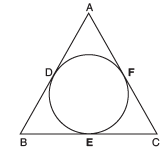Similarly,
BD = BE
and CE = CF
Since AB = AC [Given]
⇒ AB − AD = AC − AF [∵ AD = AF]
⇒ BD = CF ...(1)
But BF = BD and CF = CE
∴ From (1), we have:
BE = CE

Q14. If a, b, c are the sides of a right triangle where c is hypotenuse, prove that the radius r of the circle which touches the sides of the triangle is given by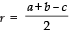Sol. Here, a, b and c are the sides of rt D ABC such that BC = a, CA = b and AB = c Let the circle touches the sides BC, CA, AB at D, E and F respectively.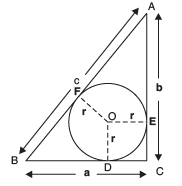= AE = AF and BD = BF
Also, CE = CD = r
∴ AF = b – r BF = a – r
Now, AB = c  ⇒  (AF + BF)
=(b – r) + (a – r)
⇒ c = b + a – 2r
⇒ 2r = a + b – cQ15. In a right Δ ABC, right angled at B, BC = 5 cm and AB = 12 cm. The circle is touching the sides of Δ ABC. Find the radius of the circle.

Sol. Let the circle with centre O and radius ‘r’ touches AB, BC and AC at P, Q, R, respectively.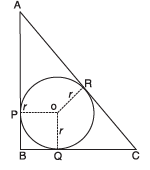Now,
AR = AP
∵ AP = AB – BP = (12 – r) cm
∴ AR = (12– r)cm
Similarly, CR = (5 – r)cm
Now, using Pythagoras theorem in rt Δ ABC, we have
AC2 = AB2 + BC2
⇒ AC2 = 122 + 52
⇒ AC = 13 cm
But AC = AR + CR = (12 – r) + (5 – r)
⇒ (12 – r) + (5 – r) = 13 cm
⇒ 17 – 2r = 13 cm
⇒ 2 r = 17 – 13 = 4 cm
⇒ r = 4/2 = 2 cm

Thus, the radius of the circle is 2 cm.

Q16. Prove that the parallelogram circumscribing a circle is a rhombus.

Sol. Since ABCD is a ║ gm
∴ AB = CD
∵ Tangents from an external point to a circle are equal,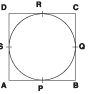⇒(AP + PB) + (RC + DR)
= (AS + DS) + (BQ + QC)
⇒ AB + CD = AD + BC
⇒ AB = AD = CD = BC
i.e., ABCD is a rhombus.

Q17. In the following figure, OP is equal to diameter of the circle. Prove that ABP is an equilateral triangle.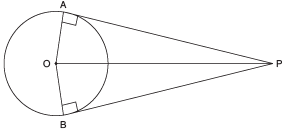Sol. Since the tangent is perpendicular to the radius through the point of contact,
∴ ∠OAP = 90°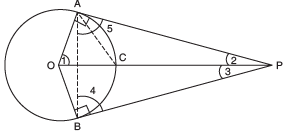Let us join AB and AC.
In right ∆OAP, OP is the hypotenuse and C is the mid point of OP.
[∵ OP is a diameter of the circle (given)]
∴ CA = CP = CO = Radius of the circle.
∴ ΔOAC is an equilateral triangle.
Since all angles in an equilateral triangle are 60°,
∴∠1 = 60°
Now, in ∆OAP, we have
∠1 + ∠OAP + ∠2 = 180°
⇒ 60° + 90° + ∠2 = 180°
⇒∠2 = 180° − 90° − 60° = 30°
Since PA and PB make equal angles with OP,
∴∠2= ∠3 ⇒∠3 = 30°
∴∠APB = ∠2 + ∠3
= 30° + 30° = 60°
Again, PA = PB.
⇒ In  ΔABP,∠4= ∠5
[Angles opposite to equal sides are equal]
Now, in ΔABP, ∠4 + ∠5 + ∠APB = 180°
⇒∠4 + ∠4 + ∠APB= 180°
⇒ 2∠4 + ∠60° = 180°
⇒ 2∠4 = 180° − 60° = 120°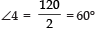Since, ∠4 = 60° ∠5 = 60°
∵ ΔABP is an equilateral Δ.
∠APB = 60°

Q18. Prove that the angle between the two tangents to a circle drawn from an external point is supplementary to the angle subtended by the line segment joining the points of contact at the centre.

Or

Two tangents PA and PB are drawn from an external point P to a circle with centre O. Prove that AOBP is a cyclic quadrilateral.

Sol.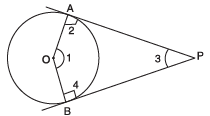We have tangents PA and PB to the circle from the external point P. Since a tangent to a circle is perpendicular to the radius through the point of contact,
∴ ∠2 = 90° and ∠4 = 90°
∠1 + ∠2 + ∠3 + ∠4 = 360°
⇒∠1 + 90° + ∠3 + 90° = 360°
⇒∠1 + ∠3 = 360° − 90° − 90°
= 180°
i.e., ∠1 and ∠3 are supplementary angles.
⇒∠ AOB and ∠APB are supplementary
⇒ AOBP is a cyclic quadrilateral.

Q19. Two equal circles, with centres O and O′, touch each other at X. OO′ produced meets the circle with centre O′ at A. AC is tangent to the circle with centre O, at the point C. O′D is perpendicular to AC. Find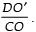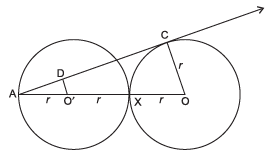Sol. AC is tangent to circle with centre O at C (given)
∠ACO = 90°  ⇒ ∆ ACO is a rt Δ
∠ADO′ = 90°   [ ä O′D ⊥ AC] ⇒ Δ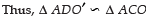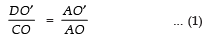But, AO′ = r,     O′X = r  and  OX = r ⇒ AO = 3r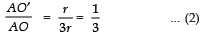From (1) and (2), we get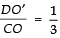Q20. Out of two concentric circles, the radius of the outer circle is 5 cm and the chord AC of length 8 cm is a tangent to the inner circle. Find the radius of the inner circle.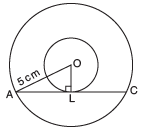Sol. Let the given chord AC of the larger circle touch the smaller circle at L. ∵ AC is a tangent at L to the smaller circle with centre O
∴ OL ⊥ AC
Also AC is a chord of the bigger circle

∴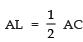Now, in rt. ΔOAL,
OL2 = OA2 – AL2

or OL2 = 52 – 42
= (5 + 4) (5 – 4)
= 9 × 1 = 9

⇒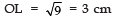Thus, the radius of the inner circle is 3 cm.

Q21. In the figure, O is the centre of a circle of radius 5cm. T is a point such that OT = 13cm and  OT intersects circle at E. If AB is a tangent to the circle at E, find the length of AB, where TP and TQ are two tangents to the circle.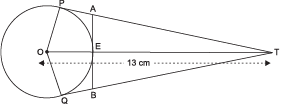Sol. O is centre of the circle and PT is a tangent to circle
∴ ∠ OPT = 90°  ⇒ ∆ OPT is a rt ∆ using Pythagoras theorem
OT2 = OP2 + PT2   or 132 = 52 + PT2
⇒ PT2 =132 – 52
⇒ PT =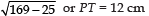Let AP = AE = x
[Tangent to a circle from an external point are equal]
⇒ AT = PT – AP = (12 – x) cm
[∵ AB is a tangent to the circle at E and OE is a radius]
∴ ∠ OEA = 90° ⇒ ∠AET  = 90°
Δ AET is a rt ∆
⇒ AT2 = AE2 + ET2
or (12 − x)2 = x2 + (13 − 5)2
⇒  144− 24x + x2 = x2 + 64 or  24x = 80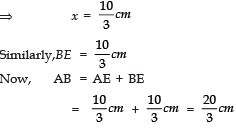Offer running on EduRev: Apply code STAYHOME200 to get INR 200 off on our premium plan EduRev Infinity!

## Mathematics (Maths) Class 10

50 videos|347 docs|138 tests

,

,

,

,

,

,

,

,

,

,

,

,

,

,

,

,

,

,

,

,

,

;